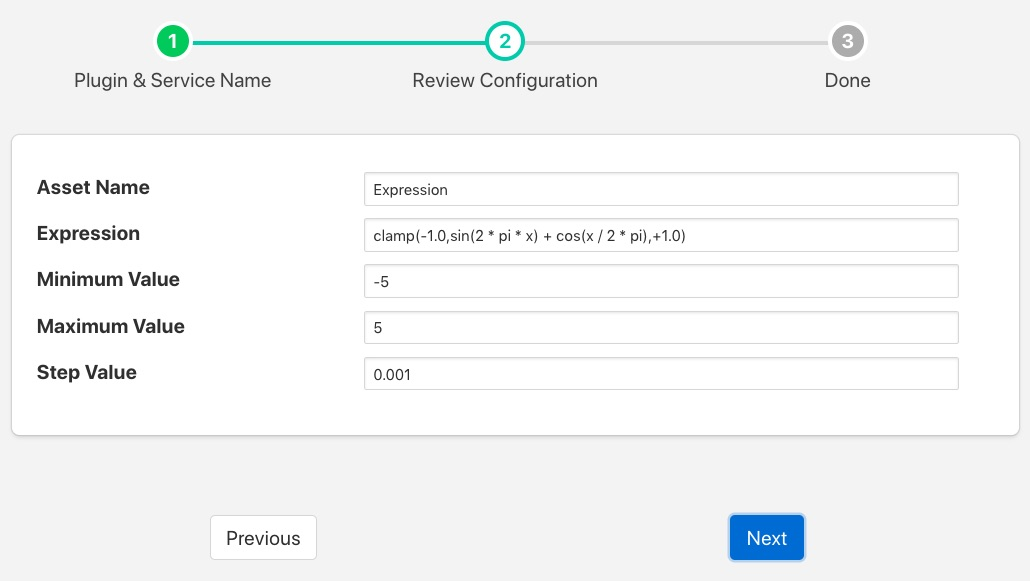# Expression South Plugin¶

The foglamp-south-expression plugin is a plugin that is used to generate synthetic data using a mathematical expression to generate data that changes over time. The user may configure the plugin with an expression of their choice and define a period in terms of samples put period of the output and the increment between each sample.The parameters that can be configured are;

• Asset Name: The name of the asset to be created inside FogLAMP.

• Expression: The expression that should be evaluated to create the asset value, see below.

• Minimum Value: The minimum value of x, where x is the value that sweeps over time.

• Maximum Value: The maximum value of x, where x is the value that sweeps over time.

• Step Value: The step in x for each call to the expression evaluation.

## Expression Support¶

The foglamp-south-expression plugin makes use of the ExprTk library to do run time expression evaluation. This library provides a rich mathematical operator set, the most useful of these in the context of this plugin are;

• Mathematical operators (+, -, *, /, %, ^)

• Functions (min, max, avg, sum, abs, ceil, floor, round, roundn, exp, log, log10, logn, pow, root, sqrt, clamp, inrange, swap)

• Trigonometry (sin, cos, tan, acos, asin, atan, atan2, cosh, cot, csc, sec, sinh, tanh, d2r, r2d, d2g, g2d, hyp)